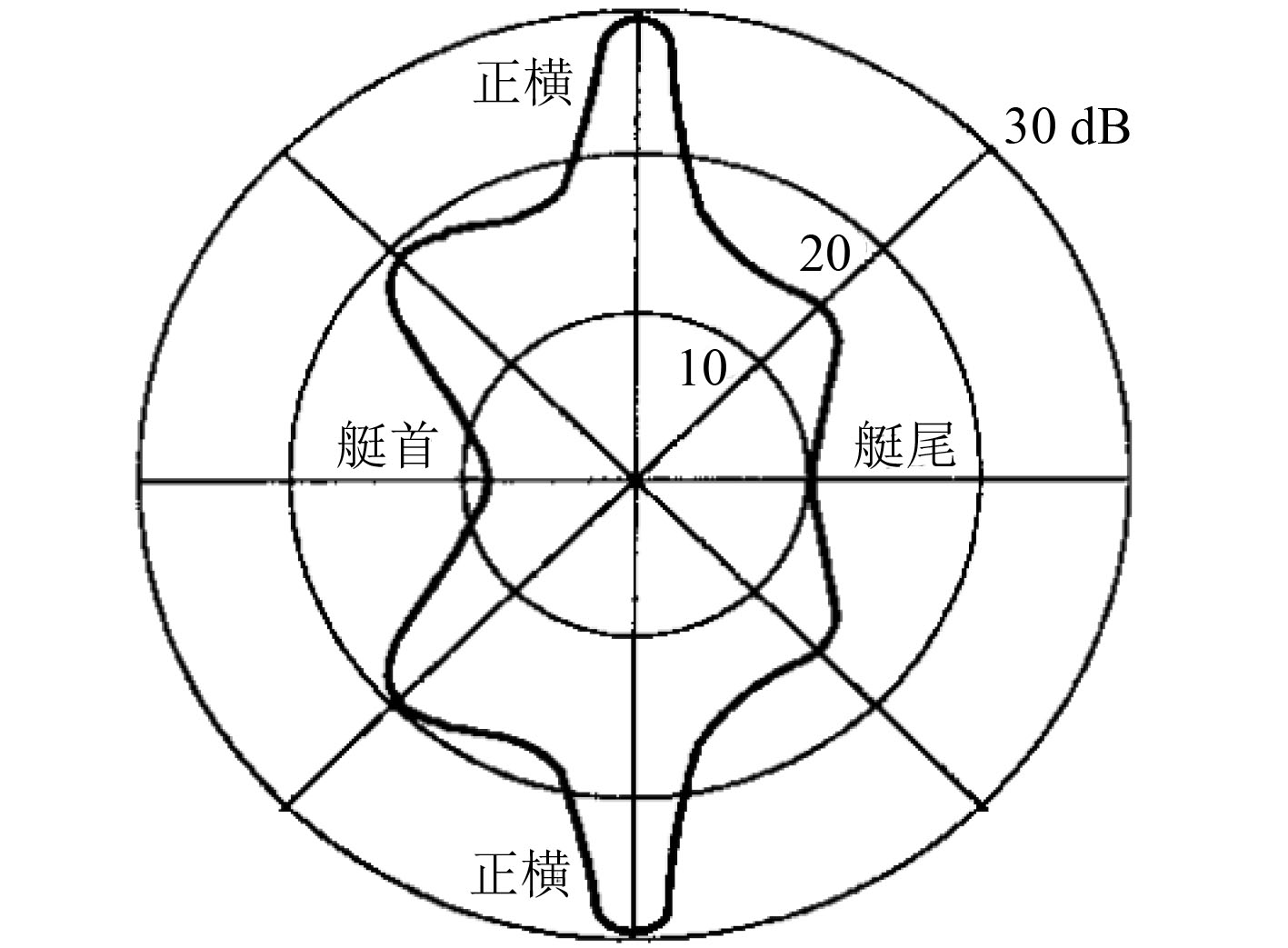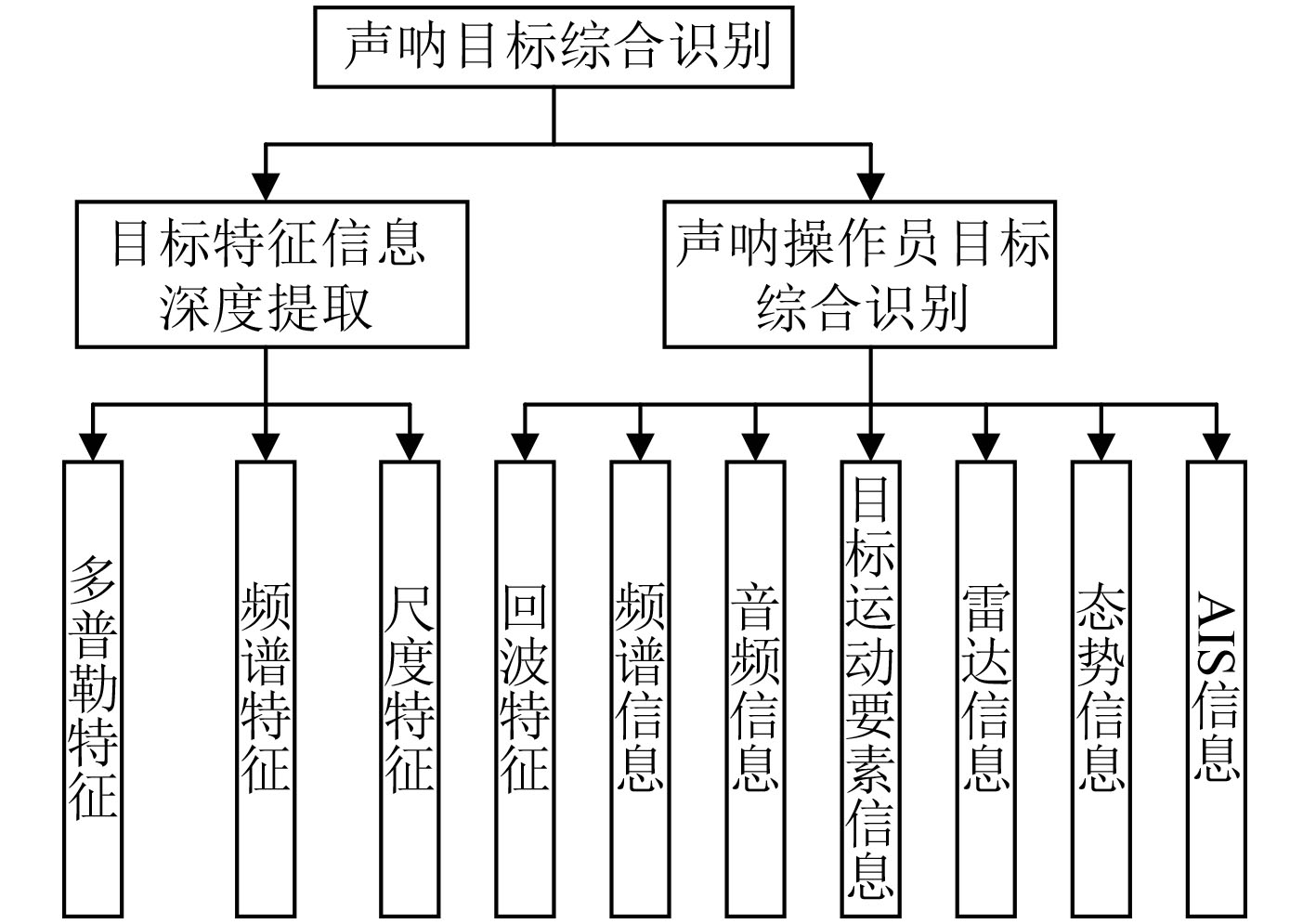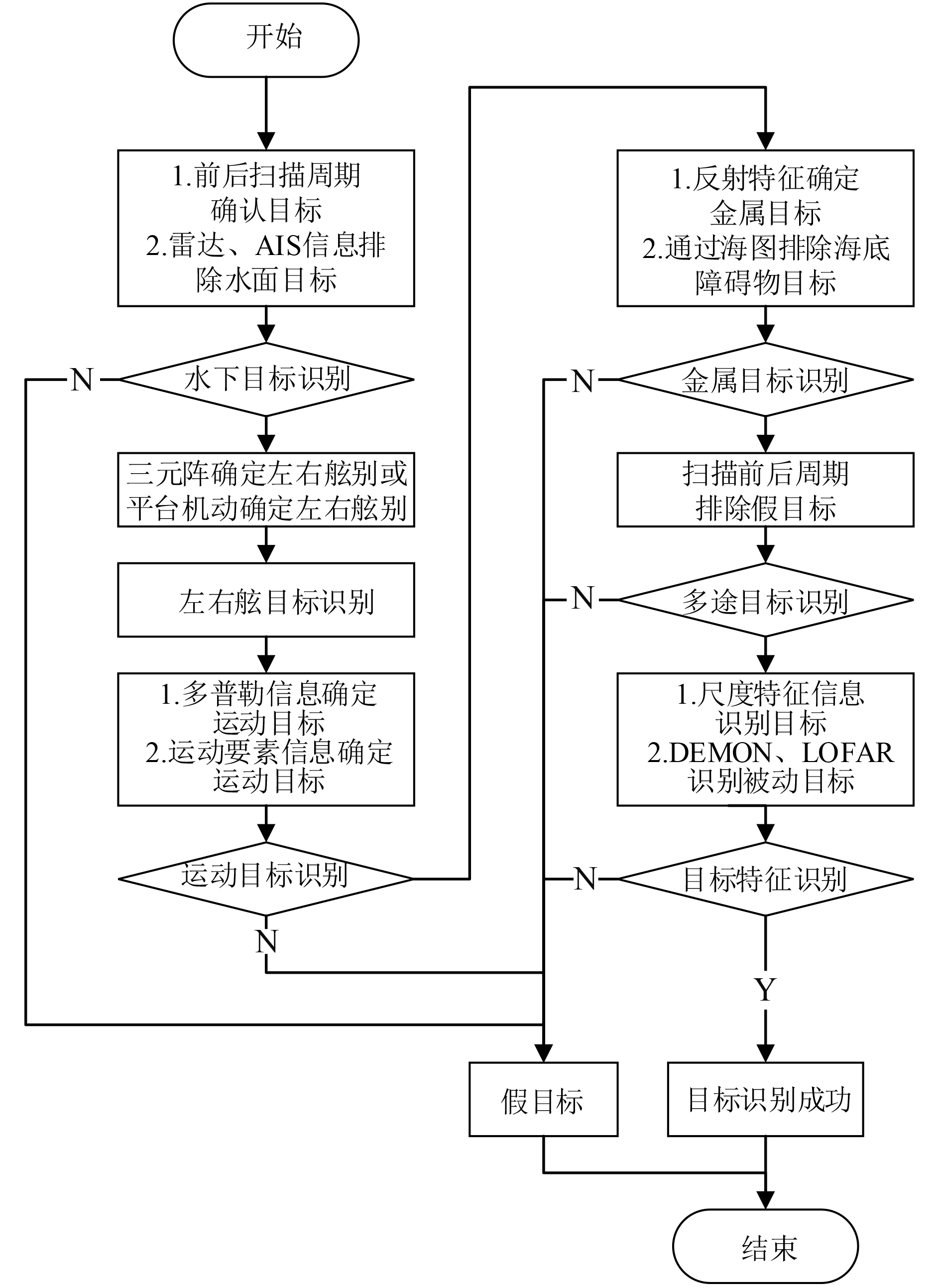﻿ 一种多源信息融合的声呐综合目标识别方法
 舰船科学技术2019, Vol. 41Issue (8): 127-130PDF

Sonar comprehensive target recognition method based on multi-source information fusion
LIANG Min-zan, MENG Hua, CHEN Yao-pei, ZENG Xiang
No. 91388 Unit of PLA, Zhanjiang 524022, China
Abstract: Aiming at the problem that the accuracy of target recognition is improved during sonar search, the characteristics of sonar target and its acquisition means are analyzed. The method of feature extraction, analysis and recognition of sonar target is studied. A sonar target synthesis with multi-information source fusion is proposed. The identification method comprehensively uses the sonar detection echo characteristic information, spectrum information, audio information, target motion element information, radar information, AIS information and other information for joint recognition, deep mining target feature information, through map characteristics, frequency domain characteristics, Multi-dimensional recognition such as listening recognition and motion elements converts the isolated and fragmented data into information advantages, forming a standard sonar target comprehensive recognition and use process, thereby improving the target recognition accuracy rate.
Key words: information fusion     target recognition     sonar
0 引　言

1 声呐目标特征

1.1 潜艇目标强度图 1 潜艇目标强度对方位角依赖性的“蝴蝶”图 Fig. 1 “Butterfly” map of submarine target strength versus azimuth dependence

 ${{TS}} = 10\lg \left( {\frac{{{{a}}{{{L}}^2}}}{{2{{\lambda}} }}} \right){\text{，}}$ (1)

 ${{TS}} = 10\lg \left( {\frac{{{{s}}{2^2}}}{{2{{\lambda}} }}} \right) + 20\lg \left( {{{{x}}^{ - 1}}\sin {{x}}} \right) + 20\lg (\cos {\rm{\theta }}){\text{。}}$ (2)

1.2 舰艇辐射噪声

 $\begin{split} & {{F}}\left[ {{{{p}}_{{T}}}({{t}}) + {{p}}({{t}})} \right] = \\ &{{F}}\left[ {\sum\nolimits_{{{n}} = - \infty }^\infty {{{{F}}_{{n}}}} {{\rm{e}}^{{\rm{jn}}\frac{{2{\text π}}}{{\rm{\tau }}}{\rm{t}}}} + {{p}}({{t}})} \right] =\\ & 2{\text π}\sum\nolimits_{n = - \infty }^\infty {{{{F}}_{{n}}}} {\rm{\delta }}\left( {{\rm{\omega }} - {{n}}\frac{{2{\text π}}}{{{T}}}} \right) + {{F}}({{\omega }}) \text{。}\end{split}$ (3)

1.3 声呐目标特性获取

1）舰艇辐射噪声特征及被动声呐系统；

2）舰艇声反射特征及主动声呐系统；

3）浅海/深海海洋环境测量。

1.4 声呐目标特征组成图 2 声呐目标识别信息源组成图 Fig. 2 Sonar target identification information source composition diagram
2 声呐目标综合识别方法

2.1 水面/水下目标识别

2.2 左右舷识别

1）本舰向左转向

2）本舰向右转向

3）对于由多途效应引起的目标方位模糊问题，则可以通过舰艇机动将目标置于舷侧波束内，然后按前述2条准则进行判决。

2.3 运动目标识别

1）潜艇的速度

 ${V_r} = ({R_1} - {R_2})/t\text{；}$ (4)

 ${V_t} = ({\varphi _2} - {\varphi _1})/t\text{；}$ (5)

 ${V_{\rm{潜}}} = \left( {{V_t} + {V_r}} \right)\text{。}$ (6)

2）潜艇反射强度

 $SL - NL - 2TL + TS + G{S_{}} + G{T_{}} = M\text{。}$ (7)

 $TS = M - G{S_{}} - G{T_{}} - SL + NL + 2TL\text{，}$ (8)
 $M = 10\lg ({A^2}/{N^2})\text{。}$ (9)

2.4 金属目标识别

2.5 目标特征识别

2.6 多途目标识别图 3 多信息源融合的声呐目标综合识别流程图 Fig. 3 Multi-information source fusion sonar target comprehensive identification flow chart

3 声呐目标特征数据采集标准

4 结　语

  闫福旺. 水声对抗技术[M]. 北京: 海洋出版社, 2003.  A.D.WAITE. 实用声纳工程(第三版)[M]北京: 电子工业出版社, 2004.  孙军平, 林建恒, 江鹏飞, 等. 舰艇水下辐射噪声谱特征传播仿真分析[J]. 声学技术, 2017, 36(5).  杨露菁, 余华. 多源信息融合理论及与用[M]. 北京: 北京邮电大学出版社, 2006.  李启虎. 数字式声纳设计原理[M]. 合肥: 安徽教育出版社, 2002.